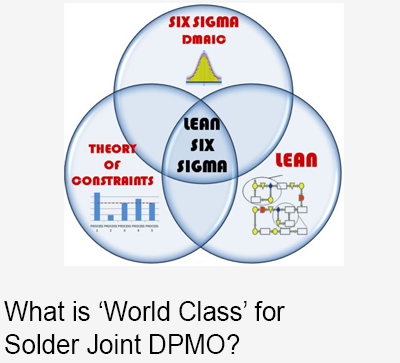The Complexity Index (Ci) is a method of scoring the manufacturing complexity of PCBA assemblies proposed by Agilent many years ago. It is a useful and accessible method of standardizing approaches to understanding complexity and therefore the appropriate manufacturing and test strategies.

Complexity Index (Ci):

Ci = ((C + J) / 100)) * D * M

Where:

C = Number of Components

J = Number of Solder Joints

D = 1 for double-sided, =.5 for single-sided

M = 1 for high mix, .5 low mixFor example, a double-sided board with 125 BOM line items might have 730 components (the sum of each BOM line item times its quantity per), 3300 solder joints, and is high mix (say less than 10,000 per run size).  Its Ci would be:

((730 + 3330) / 100 * 1 * 1 = 40.6

 Complexity Index Complexity Class <25 Low >25 and <75 Medium >75 High

## SUBSCRIBE NOW